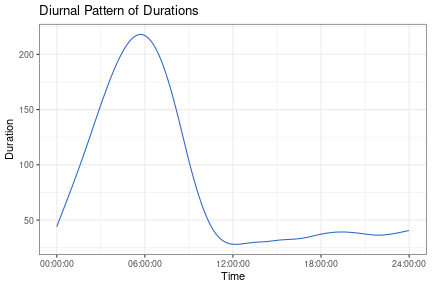# Case Study: Bookshop Orders

## Introduction

We loosely follow Tomanová and Holý (2021) and analyze the timing of orders from a Czech antiquarian bookshop. Besides seasonality and diurnal patterns, one would expect the times of orders to be independent of each other. However, this is not the case and we use a GAS model to capture dependence between the times of orders.

A strand of financial econometrics is devoted to analyzing the timing of transactions by the so-called autoregressive conditional duration (ACD) model introduced by Engle and Russell (1998). For a textbook treatment of such financial point processes, see e.g. Hautsch (2012).

library(dplyr)
library(tidyr)
library(ggplot2)
library(gasmodel)

## Data Preparation

Let us prepare the analyzed data. We use data bookshop_sales containing times of orders from June 8, 2018 to December 20, 2018. We calculate differences of subsequent times, i.e. durations. To avoid zero durations, we set them to 0.5 second.

data(bookshop_sales)
data_dur <- bookshop_sales %>%
mutate(duration = as.numeric(time - lag(time)) / 60) %>%
mutate(duration = recode(duration, "0" = 0.5)) %>%
mutate(just_time = as.vector(as.POSIXct(format(time, "1970-01-01 %H:%M:%S"), tz = "UTC"))) %>%
drop_na()

There is a strong diurnal pattern present, as we can see from the following figure:

model_spline <- smooth.spline(data_dur$just_time, data_dur$duration, df = 10)

ggplot(mapping = aes(x = model_spline$x, y = model_spline$y)) +
labs(title = "Diurnal Pattern of Durations", x = "Time", y = "Duration") +
geom_line(color = "#2E67C5") +
scale_x_time(breaks = seq(from = 0, to = 24 * 60 * 60, by = 6 * 60 * 60)) +
theme_bw()We adjust the observed durations for this diurnal pattern and extract the time series to be analyzed:

data_dur <- data_dur %>%
mutate(duration_spline = predict(model_spline, x = just_time)$y) %>% mutate(duration_adj = duration / duration_spline) %>% select(-just_time) y <- data_dur$duration_adj

## Model Estimation

distr(filter_type = "duration", filter_dim = "uni", filter_default = TRUE)[, 1:3]
#>          distr_title param_title    distr
#> 5        Exponential       Scale      exp
#> 7              Gamma       Scale    gamma
#> 9  Generalized Gamma       Scale gengamma
#> 24           Weibull       Scale  weibull

First, we estimate the model based on the exponential distribution:

est_exp <- gas(y = y, distr = "exp")
est_exp
#> GAS Model: Exponential Distribution / Scale Parametrization / Unit Scaling
#>
#> Coefficients:
#>                      Estimate  Std. Error   Z-Test  Pr(>|Z|)
#> log(scale)_omega  -0.00085202  0.00114896  -0.7416    0.4584
#> log(scale)_alpha1  0.04888439  0.00650562   7.5142 5.727e-14 ***
#> log(scale)_phi1    0.96343265  0.00910508 105.8126 < 2.2e-16 ***
#> ---
#> Signif. codes:  0 '***' 0.001 '**' 0.01 '*' 0.05 '.' 0.1 ' ' 1
#>
#> Log-Likelihood: -5608.518, AIC: 11223.04, BIC: 11243.01

Second, we estimate the model based on the Weibull distribution:

est_weibull <- gas(y = y, distr = "weibull", par_static = c(FALSE, TRUE))
est_weibull
#> GAS Model: Weibull Distribution / Scale Parametrization / Unit Scaling
#>
#> Coefficients:
#>                     Estimate Std. Error   Z-Test  Pr(>|Z|)
#> log(scale)_omega  -0.0019175  0.0013552  -1.4149    0.1571
#> log(scale)_alpha1  0.0562619  0.0082010   6.8604 6.867e-12 ***
#> log(scale)_phi1    0.9622643  0.0102230  94.1278 < 2.2e-16 ***
#> shape              0.9442209  0.0094299 100.1300 < 2.2e-16 ***
#> ---
#> Signif. codes:  0 '***' 0.001 '**' 0.01 '*' 0.05 '.' 0.1 ' ' 1
#>
#> Log-Likelihood: -5591.442, AIC: 11190.88, BIC: 11217.51

Third, we estimate the model based on the gamma distribution:

est_gamma <- gas(y = y, distr = "gamma", par_static = c(FALSE, TRUE))
est_gamma
#> GAS Model: Gamma Distribution / Scale Parametrization / Unit Scaling
#>
#> Coefficients:
#>                    Estimate Std. Error   Z-Test  Pr(>|Z|)
#> log(scale)_omega  0.0013296  0.0013395   0.9926    0.3209
#> log(scale)_alpha1 0.0518896  0.0071672   7.2399 4.491e-13 ***
#> log(scale)_phi1   0.9634327  0.0093853 102.6532 < 2.2e-16 ***
#> shape             0.9420850  0.0153854  61.2325 < 2.2e-16 ***
#> ---
#> Signif. codes:  0 '***' 0.001 '**' 0.01 '*' 0.05 '.' 0.1 ' ' 1
#>
#> Log-Likelihood: -5601.735, AIC: 11211.47, BIC: 11238.1

Fourth, we estimate the model based on the generalized gamma distribution:

est_gengamma <- gas(y = y, distr = "gengamma", par_static = c(FALSE, TRUE, TRUE))
est_gengamma
#> GAS Model: Generalized Gamma Distribution / Scale Parametrization / Unit Scaling
#>
#> Coefficients:
#>                    Estimate Std. Error  Z-Test  Pr(>|Z|)
#> log(scale)_omega  -0.049164   0.018903 -2.6009  0.009299 **
#> log(scale)_alpha1  0.069834   0.011670  5.9841 2.176e-09 ***
#> log(scale)_phi1    0.951761   0.015024 63.3493 < 2.2e-16 ***
#> shape1             1.764362   0.150759 11.7032 < 2.2e-16 ***
#> shape2             0.682971   0.033690 20.2723 < 2.2e-16 ***
#> ---
#> Signif. codes:  0 '***' 0.001 '**' 0.01 '*' 0.05 '.' 0.1 ' ' 1
#>
#> Log-Likelihood: -5562.092, AIC: 11134.18, BIC: 11167.47

By comparing the Akaike information criterion (AIC), we find that the most general model, i.e. the one based on the generalized gamma distribution, is the most suitable.

AIC(est_exp, est_weibull, est_gamma, est_gengamma)
#>              df      AIC
#> est_exp       3 11223.04
#> est_weibull   4 11190.88
#> est_gamma     4 11211.47
#> est_gengamma  5 11134.18